Welcome to ...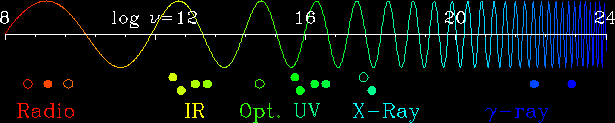# The MultiWavelength Sky

Web Page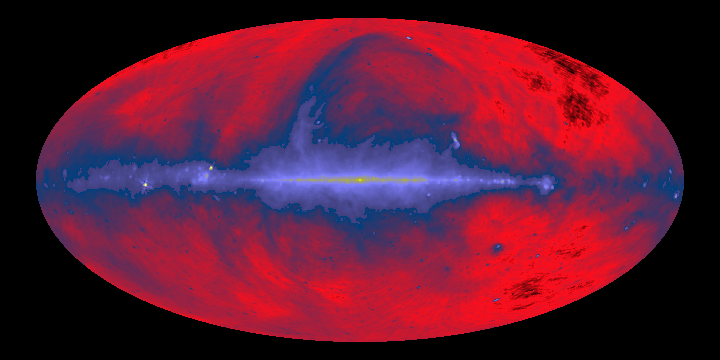Credit: Max Planck Institute for Radio Astronomy, generated by Glyn Haslam
Comment: This survey is a mosaic of data taken at Jodrell Bank, Effelsberg and Parkes telescopes. The data was distributed in the "NRAO Images from the Radio Sky" CD ROM. This image was generated by SkyView.
Energy= 1.69x10^-6 eV Frequency= 408x10^6 Hz (408MHz) Wavelength= 73.5 cm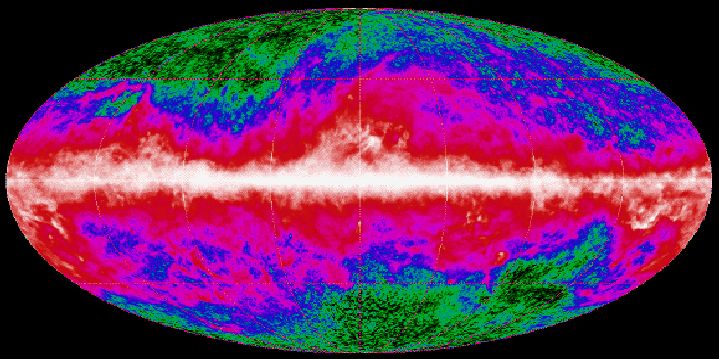Credit: NASA Goddard Space Flight Center, Cosmic Background Explorer (COBE) Project
Comment: This image was created using data from the COBE Diffuse Infrared Background Experiment (DIRBE) and represents the annual average map at 240 microns.
Energy= 5.18x10^-3 eV Frequency= 1.25x10^12 Hz Wavelength= 2.40x10^-2 cm (240 microns)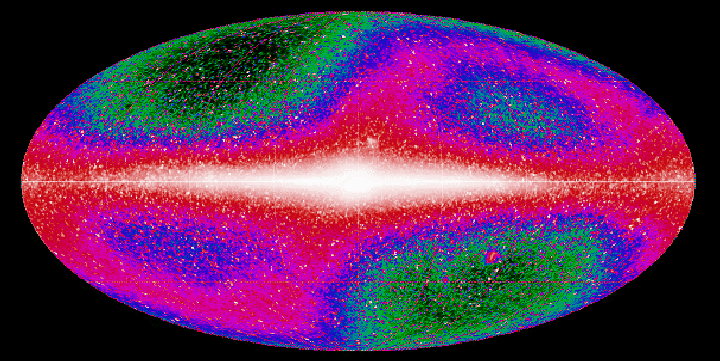Credit: NASA Goddard Space Flight Center, Cosmic Background Explorer (COBE) Project
Comment: This image was created using data from the COBE Diffuse Infrared Background Experiment (DIRBE) and represents the annual average map at 3.5 microns.
Energy= 0.36 eV Frequency= 8.6x10^13 Hz Wavelength= 3.5x10^-4 cm(3.5 microns)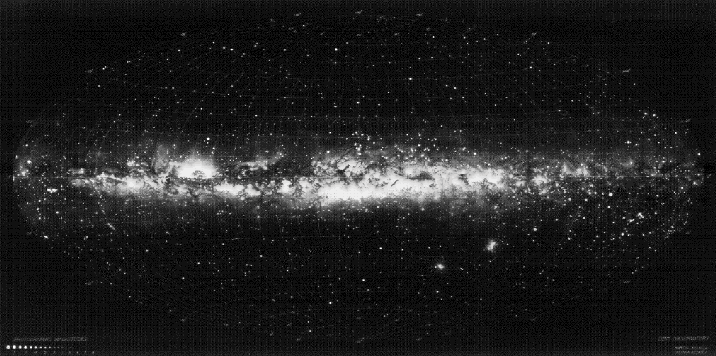Credit: Lund Observatory
Comment: This all-sky panoramic image was created under the direction of Knut Lundmark at Lund Observatory in the 1940s.
Energy= 2.5 eV Frequency= 6.0x10^14 Hz Wavelength= 5.0x10^-5 cm(5000 Angstroms)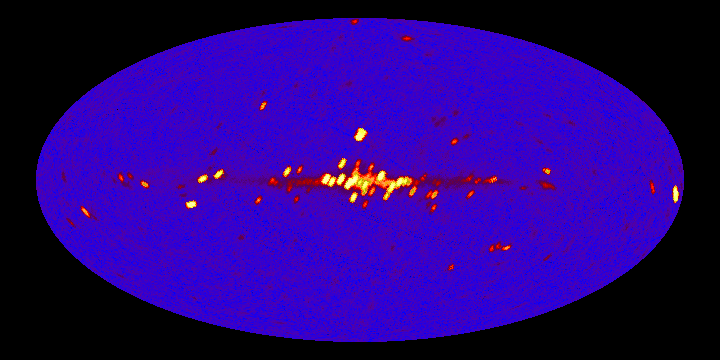Credit: NASA Goddard Space Flight Center, original map generated by Keith Jahoda.
Comment:This all-sky map was made from roughly six months of scanning data from the A2 instrument on board the HEAO-1 satellite. This image was generated by SkyView.
Energy= 2keV - 10keV Frequency= 5x10^17 Hz - 2.5x10^18 Hz Wavelength= 6.2x10^-8 cm - 1.2x10^-8 cm (6.2 - 1.2 angstroms)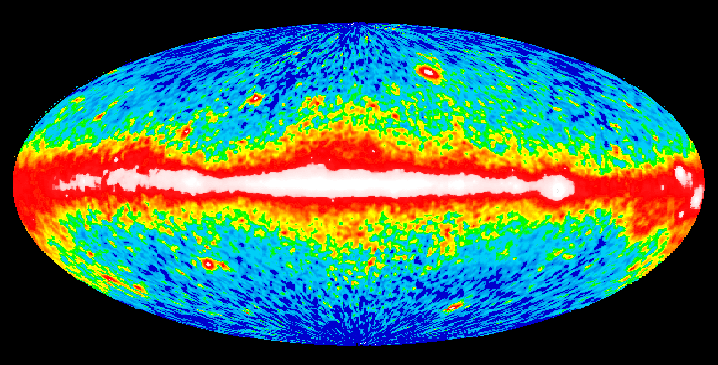Credit: NASA Goddard Space Flight Center, Compton Gamma-Ray Observatory (CGRO)
Comment: This map was generated by the EGRET (Energetic Gamma-Ray Experiment Telescope) team at Goddard using EGRET data from the first year of CGRO operations (beginning April 1991). It represents the first all-sky survey at energies above 100keV.
Energy= > 100MeV Frequency= > 2.5x10^22 Hz Wavelength= < 1.2 x 10^-12 cm ( < 0.00012 angstroms )